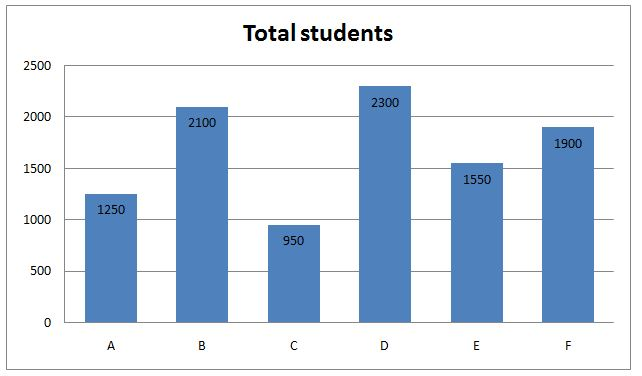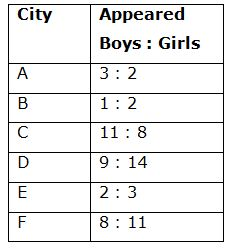# NIACL AO Mains– Quantitative Aptitude Questions Day- 05

Dear Readers, Bank Exam Race for the Year 2019 is already started, To enrich your preparation here we have providing new series of Practice Questions on Quantitative Aptitude – Section. Candidates those who are preparing for NIACL AO Mains 2019 Exams can practice these questions daily and make your preparation effective.

[WpProQuiz 5089]

Directions (Q. 1 – 5): Find the wrong term in the following number series?

1) 12, 6, 9, 22.5, 77.75, 354.375

a) 22.5

b) 354.375

c) 77.75

d) 9

e) 12

2) 254, 303, 815, 895, 1896, 2017

a) 895

b) 1896

c) 2017

d) 254

e) 303

3) 1182, 591, 1773, 354.4, 2482.2

a) 1182

b) 591

c) 1773

d) 4

e) 2482.2

4) 853, 880, 988, 1160, 1380, 1638

a) 1638

b) 1380

c) 1160

d) 988

e) 853

5) 1123, 1119, 1109, 1081, 997, 755

a) 1119

b) 1109

c) 755

d) 1123

e) 997

Directions (Q. 6 – 10): Study the following information carefully and answer the given questions:

The following bar graph shows the total number of students appeared for the certain examination from different cities and the table shows the ratio between the total number of boys to that of girls appeared for the examination.6) Find the difference between the total number of boys passed the examination from city C to that of total girls passed the examination from city F, if the percentage of students passed from city C and F is 64 % and 72 % respectively and the ratio between the boys to that of girls passed the examination from city C is 5 : 3 and the percentage of boys passed the examination from city F is 50 %?

a) 248

b) 216

c) 304

d) 352

e) None of these

7) Total appeared boys in city A and B together is what percentage of the total appeared boys in city C and D together?

a) 100 %

b) 120 %

c) 80 %

d) 55 %

e) None of these

8) Find the ratio between the total number of girls passed from city E to that of total number of students passed from city C, if the percentage of students passed from city E is 56 % and the ratio of total number of girls to that of boys passed the examination from city E is 9 : 5 and the total number of girls passed from city C is 342, which is equal to 50 % of the total number of students passed from city C?

a) 51: 117

b) 155: 342

c) 19: 59

d) 29: 73

e) None of these

9) Find the average number of appearing students from all the given cities together?

a) 1450

b) 1625

c) 1575

d) 1850

e) None of these

10) Total number of boys appeared for the examination from city A, C and E together is approximately what percentage more/less than the total number of girls appeared for the examination from city B, D and F together?

a) 43 %

b) 74 %

c) 35 %

d) 51 %

e) 66 %

Directions (Q. 1 – 5):

The correct series is,

12, 6, 9, 22.5, 78.75, 354.375

The pattern is, ×0.5, ×1.5, ×2.5, ×3.5, ×4.5,…

The wrong term is, 77.75

The correct series is,

254, 303, 815, 896, 1896, 2017

The pattern is, +72, +83, +92, +103, +112,….

The wrong term is, 895

The correct series is,

1182, 591, 1773, 354.6, 2482.2

The pattern is, ÷ 2, *3, ÷ 5, *7,… (Prime no’s)

The wrong term is, 354.4

The correct series is,

The difference of difference is, 92, 82, 72, 62,…

The wrong term is, 1380

The correct series is,

1123, 1119, 1109, 1081, 999, 755

The pattern is, -(31 +1), -(32 +1), -(33 +1), -(34 +1), -(35 +1),…

The wrong term is, 997

Directions (Q. 6 – 10):

The total number of boys passed the examination from city C

= > 950*(64/100)*(5/8) = 380

The total number of girls passed the examination from city F

= > 1900*(72/100)*(50/100) = 684

Required difference = 684 – 380 = 304

Total appeared boys in city A and B together

= > 1250*(3/5) + 2100*(1/3)

= > 750 + 700 = 1450

Total appeared boys in city C and D together

= > 950*(11/19) + 2300*(9/23)

= > 550 + 900 = 1450

Required % = (1450/1450)*100 = 100 %

The total number of girls passed from city E

= > 1550*(56/100)*(5/14) = 310

The total number of students passed from city C

= > 342*2 = 684

Required ratio = 310: 684 = 155: 342

The total number of appearing students from all the given cities together

= > 1250 + 2100 + 950 + 2300 + 1550 + 1900 = 10050

Required average = 10050/6 = 1675

Total number of boys appeared for the examination from city A, C and E together

= > 1250*(3/5) + 950*(11/19) + 1550*(2/5)

= > 750 + 550 + 620 = 1920

Total number of girls appeared for the examination from city B, D and F together

= > 2100*(2/3) + 2300*(14/23) + 1900*(11/19)

= > 1400 + 1400 + 1100 = 3900

Required % = [(3900 – 1920)/3900]*100 = 51 %